# 基于滑移速度壁模型的复杂边界湍流大涡模拟1)

(中国科学院力学研究所非线性力学国家重点实验室，北京 100190);(中国科学院大学工程科学学院，北京 100049)

# LARGE-EDDY SIMULATION OF FLOWS WITH COMPLEX GEOMETRIES BY USING THE SLIP-WALL MODEL1)

Shi Beiji, He Guowei, Wang Shizhao2)LNM, Institute of Mechanics, Chinese Academy of Sciences, Beijing 100190, China;School of Engineering Sciences, University of Chinese Academy of Sciences, Beijing, China

Abstract

A slip-wall model is combined with the immersed boundary method for large-eddy simulation of flows with complex geometries. Firstly, large eddy simulation of flows over periodic hills are conducted to evaluate the effects of the tangential pressure gradient in wall model. Both the equilibrium stress balance model which based on the assumption of an equilibrium boundary-layer and the non-equilibrium wall model, in which the pressure gradient blend into the simplified thin boundary-layer equations and the RANS-like eddy viscosity in both the procedure of computing the wall-shear stress and reconstructing the wall-slip velocity, are utilized for comparison. The numerical results show that the pressure coefficient is not sensitive to the types of wall model which we considered, especially the strong pressure gradient in front of the hill crest is well catched by both models. However, when taking into account the tangential pressure gradient, the non-equilibrium wall model is superior to the equilibrium one for its ability to improve the prediction of the wall-shear stress and flow separation. When the equilibrium stress balance model is used, the wall-shear stress is heavily under-predicted and remarkable discrepancies of the mean velocity profiles can also be seen in the recirculation region. By comparison, the correction of the non-equilibrium wall model is proportional to both the tangential pressure gradient and the normal distance away from the wall, thus the hydrodynamic coefficients and the mean flow statistics are all in good agreement with the references even on very coarse grids. Secondly, large-eddy simulation of flow around an axisymmetric body is conducted to assess the applicability of current method when applied to high Reynolds number wall-bounded turbulent flows. The flow structures and the hydrodynamic characteristics are well predicted by the non-equilibrium wall model. This work confirms that the immersed boundary method in combination with the non-equilibrium slip-wall model is a possible and promising way to deal with turbulent flows which have complex geometries.

Keywords： slip-wall model ; immersed boundary method ; large-eddy simulation ; pressure gradient ; flow over periodic hills ; flow around an axisymmetric body

0

Shi Beiji, He Guowei, Wang Shizhao. LARGE-EDDY SIMULATION OF FLOWS WITH COMPLEX GEOMETRIES BY USING THE SLIP-WALL MODEL1)[J]. Chinese Journal of Theoretical and Applied Mechanics, 2019, 51(3): 754-766 https://doi.org/10.6052/0459-1879-19-033

## 1 数值方法

### 1.1 控制方程及其离散

$$\frac{\partial \tilde {u}_i }{\partial x_i } = 0\tag{1}\\$$

$$\frac{\partial \tilde {u}_i }{\partial t} + \frac{\partial \tilde {u}_i \tilde {u}_j }{\partial x_j } = - \frac{1}{\rho }\frac{\partial \tilde {p}}{\partial x_i } + \nu \frac{\partial ^2\tilde {u}_i }{\partial x_j \partial x_j } - \frac{\partial \tilde {\tau }_{ij} }{\partial x_j }\mbox{ + }f_i\tag{2}$$

$$\nu _t = C_{\rm w}^2 \bar {\varDelta }^2\frac{\left( {\tilde {S}_{ij}^d \tilde {S}_{ij}^d } \right)^{3 / 2}}{\left( {\tilde {S}_{ij} \tilde {S}_{ij} } \right)^{5 / 2} + \left( {\tilde {S}_{ij}^d \tilde {S}_{ij}^d } \right)^{5 / 4}}\tag{4}$$

$$\tilde {S}_{ij}^d = \frac{1}{2}\left( {\tilde {g}_{ij}^2 + \tilde {g}_{ji}^2 } \right) - \frac{1}{3}\delta _{ij} \tilde {g}_{kk}^2 \tag{5}$$

$$\tilde {g}_{ij} = \frac{\partial \tilde {u}_i }{\partial x_j }\tag{6}$$

$$\frac{\tilde {u}_i^{\ast } - \tilde {u}_i^n }{\Delta t} = rhs^{n + 1 / 2} - \frac{\partial \tilde {p}^n}{\partial x_i } + f_i^{n + 1 / 2}\tag{7}$$

$$\tilde {u}_i^{n + 1} = \tilde {u}_i^\ast - \Delta t\frac{\partial \delta p}{\partial x_i }\tag{8}$$

$$\frac{\partial ^2\delta p}{\partial x_i \partial x_i } = \frac{1}{\Delta t}\frac{\partial \tilde {u}_i^\ast }{\partial x_i }\tag{9}$$

$$\tilde {p}^{n + 1} = \tilde {p}^n + \delta p\tag{10}$$

### 1.2 浸入边界方法

\begin{equation} \label{eq11} f_i^{n + 1 / 2} = W_{ij} F_j^{n + 1 / 2} , {i,j = 1,2,3} \tag{11} \end{equation}

\begin{equation} \label{eq12} F_j^{n + 1 / 2} = \frac{U_j^{\rm b} - \hat {U}_j^{\ast {\ast }} }{\Delta t}, {j = 1,2,3} \tag{12} \end{equation}

$为该网格上的预估速度并可以由其周围欧拉网格上的速度插值得到 \begin{equation} \label{eq13} \hat {U}_j^{\ast {\ast }} = W_{ji} \hat {u}_i^{\ast {\ast }} ,\quad {i,j = 1,2,3}\tag{13} \end{equation} 其中,$\hat {u}_i^{\ast{\ast }}$为欧拉网格上的预估速度,由方程(7)在不考虑体积力$f_i^{n + 1 / 2}$时计算得到 \begin{equation} \label{eq14} \hat {u}_i^{\ast {\ast }} = \tilde {u}^n + \Delta t\left( {rhs^{n + 1 / 2} - \frac{\partial \tilde {p}^n}{\partial x_i }} \right)\tag{14} \end{equation} ## 2 浸入边界方法与壁模型的结合 以壁面滑移速度的形式将大涡模拟壁模型与浸入边界方法相结合.由于壁模型主要用于计算壁面切应力和重构壁面滑移速度,而流场计算的欧拉网格与几何边界通常并不重合,因此将模型方程以及流动物理量投影到局部正交的坐标系下.近壁区流动速度${ {{u}}}$可以在该局部坐标系下沿切向和法向分解为 $${ {{u}}} = { {{u}}}_{{\rm w}} + u_\eta { {{e}}}_{{\eta}} + u_\xi { {{e}}}_{{\xi }}\tag{15}$$ 其中${ {{e}}}_{{\eta}} $和${ {{e}}}_{{\xi }} $分别为局部坐标系下的法向和切向单位矢量,$u_\eta $和$u_\xi $分别为局部的法向和切向速度. 文中主要研究几何边界为静止状态的流动问题,因此边界的运动速度${ {{u}}}_{{\rm w}} $设置为零. 采用如下方式构造壁面滑移速度来计算等效体积力.该方法在湍流近壁区内维持切向速度的线性分布并同时满足流动控制体内的积分动量守恒.壁面上的切向速度$u_{\xi ,{\rm S}} $和法向速度$u_{\eta ,{\rm S}}$可以分别表示为 $$u_{\xi ,{\rm S}} = u_{\xi ,{\rm P}} - \frac{\delta _{\rm P} }{\left( {\nu \mbox{ + }\nu _{\rm t} } \right)}\left( {\frac{\tau _{\rm w} }{\rho } + S\delta _{\rm P} } \right)\tag{16}$$ $$u_{\eta ,{\rm S}} = 0\tag{17}$$ 式(16)中$u_{\xi ,{\rm P}}$为壁模型与大涡模拟交界面(本文定义为匹配面)上的切向速度.$u_{\xi,{\rm P}} $可从大涡模拟的流场在匹配面上插值得到.$\delta _{\rm P}$为匹配面距离壁面的法向距离,$\nu _{\rm t} $为涡黏系数.右端项$S$为薄边界层方程中的非平衡项(见式(18)).该项的具体形式以及壁面摩擦力$\tau _{\rm w}$的求解过程将在以下内容中进行详细介绍.本文中匹配面距离壁面的法向距离设置为$2\Delta h(\Delta h$为欧拉网格宽度),而移动最小二乘插值的模板半宽为$1.2\Delta h$.因此在匹配面上的插值操作并不涉及到边界内部的固体点,并同时可以避免在边界和匹配面插值的相互干扰. 本文采用Duprat等提出的壁模型来计算壁面切应力,从而重构壁面滑移速度.在局部坐标系下,边界层内区流动的切向动量方程为[17-19] \begin{equation} \label{eq16} \frac{\partial }{\partial \eta }\left[ {\left( {\nu \mbox{ + }\nu _{\rm t} } \right)\frac{\partial { { {u}}}}{\partial \eta } \cdot { { {e}}}_{{\xi}} } \right] = S\tag{18} \end{equation} 其中,$S = \dfrac{1}{\rho }\nabla \bar {P}_{\rm m} \cdot { {{e}}}_{{\xi}} + \dfrac{\partial { {{u}}}}{\partial t} \cdot{ {{e}}}_{{\xi }} + \left( {{ { {u}}} \cdot \nabla }\right){ {{u}}} \cdot { {{e}}}_{{\xi }} $,$\bar {P}_{\rm m}$表示匹配面上的滤波压力,可以由大涡模拟插值得到并且在边界层内区沿法向保持为常数. 方程(18)是关于切向速度的偏微分方程,可以在边界层内区直接求解,其中下壁面满足无滑移边界条件,上边界的边界条件则由大涡模拟提供.仿照Wang和Moin的工作,本文主要考虑模型方程的两种简化形式： (1)$S = 0$,对应于平衡层模型(文中标记为EB模型); (2)$S = 1 / \rho \left( {\nabla \bar {P}_{\rm m} \cdot { {{e}}}_{{ \xi }} } \right)$,即右端项仅考虑切向压力梯度引起的非平衡效应(文中标记为NEB模型). 在这两种情况下,将动量方程沿法向积分可以得到壁面摩擦力的解析表达式 \begin{equation} \label{eq17} \tau _{\rm w} = {\rho }\left( {u_{\xi ,{\rm P}} - S\int_0^{\delta _{\rm P} } {\frac{\eta {\rm d}\eta }{\nu + \nu _{\rm t} }} } \right)\bigg/ {\int_0^{\delta _{\rm P} } {\frac{{\rm d}\eta }{\nu + \nu _{\rm t} }} }\tag{19} \end{equation} Duprat 等 对van Driest公式进行修正,以考虑切向压力梯度对近壁涡黏的影响 $$\frac{\nu_{\rm t}}{\nu } = \kappa \eta ^\ast \left[ {\alpha + \eta ^\ast \left( {1 - \alpha } \right)^{\frac{3}{2}}} \right]^\beta \left( {1 - {\rm e}^{ - \frac{\eta ^\ast }{1 + \alpha ^3A}}} \right)^2\tag{20}$$ 其中壁面单位定义为$\eta ^\ast = \eta u_{\tau {p}} / \nu (\eta $为距离壁面的法向距离). 特征速度$u_{\rm \tau p}= \sqrt {u_\tau ^2 + u_{\rm p}^2 } $,其中$u_\tau$为摩擦速度,$u_{\rm p} = \left| {\left( {\nu / \rho }\right)\left( {\nabla \bar {P}_{\rm m} \cdot { {{e}}}_{ { \xi}} } \right)} \right|^{1 / 3}$用于表征切向压力梯度的影响.参数$\alpha = u_\tau ^2 / u_{\rm\tau p}^2$可以定量衡量壁面切应力与切向压力梯度的相对大小.模型参数设置为$\kappa = 0.41$,$\beta = 0.78$,$A = 18$20. 引入上述形式的涡黏衰减函数,方程(19)是关于壁面切应力的超越方程.为便于求解,在计算当前时刻的壁面切应力时,右端项的涡黏系数则根据方程(20)利用上一时间步的壁面切应力来计算. 上述形式的壁模型最终被简化为代数模型.由该模型计算壁面切应力以重构壁面滑移速度,并由此构建等效体积力,从而实现大涡模拟壁模型与浸入边界方法的结合. ## 3 结果与讨论 本文分别采用NEB模型$\bigg(S = 1 / \rho \left( {\nabla \bar{P}_{\rm m} \cdot { {{e}}}_{ { \xi }} }\right)\bigg)$以及EB模型($S = 0,\alpha =1)$,在周期山状流和回转体绕流中实现了浸入边界方法与滑移速度壁模型相结合的大涡模拟,并通过不同模型计算结果的对比考查了在构造壁模型时考虑切向压力梯度的作用. ### 3.1 周期山状流 本文通过周期山状流的大涡模拟考查在壁模型中考虑压力梯度的作用.该流动具有复杂的几何边界,流动沿流向具有压力梯度并且伴随分离、再附等复杂的流动现象,是验证大涡模拟壁模型的标准算例.Breuer等 对该流动进行了详尽的实验和数值模拟研究.他们通过PIV实验对宽广雷诺数范围内的周期山状流进行定量的测量分析,并进一步的采用基于贴体网格的直接数值模拟以及壁面解析的大涡模拟对该雷诺数范围内的流动进行细致的研究.他们的数值和实验对比结果被广泛用作周期山状流数值模拟的参考数据库.本文选择了其中雷诺数最高的一个算例为本文大涡模拟的参考对象. 大涡模拟的雷诺数$Re_h = U_{\rm b} h / \nu = 10~595$,其中$U_{b} $和$h$分别为整体流动的平均速度以及山峰高度.图1中给出了周期山状流的几何构型与计算域的示意图,流向、法向和展向的计算域分别为$L_x= 9h$,$L_y = 4h$以及$L_z = 4.5h$.其中,槽道的最大高度为$3.035h$,计算域法向边界的位置根据网格尺度进行调整以保证与欧拉网格不重合.流场的计算在两种不同尺度的均匀笛卡尔网格上进行,其中细网格宽度$\Delta h = h / 32$,该网格尺度与Temmerman 等壁面模化大涡模拟中的近壁贴体网格尺度一致. 粗网格的宽度$\Delta h =h /16$,该算例的总网格量仅为Breuer等壁面解析大涡模拟的5{％}.以壁面单位无量纲化后的欧拉网格尺度以及匹配面的位置沿山状壁面的分布见图2.注意文中匹配面距离壁面的法向距离设置为$\delta _{\rm p} = 2\Delta h$,在粗网格下无量纲化后的匹配面位置最远已经达到$\delta _{\rm p}^+ \approx 160$. 计算过程中的壁模型同时施加在山状流的上、下壁面.流向及展向均设置为周期性边界条件,计算域的法向边界则为自由滑移边界条件.周期山状流采用定流量的方式驱动,即在流场每一瞬时根据入口截面的流量确定相应的压力梯度,并将其施加于全场以驱动流体运动.本文在施加NEB模型时考虑了该驱动力的影响,模型中的压力梯度为流场计算得到的压力梯度与瞬时流场的驱动压力梯度之和.数值模拟中的初始流场发展30个"流过"时间,物理量的统计时间则为50个"流过"时间.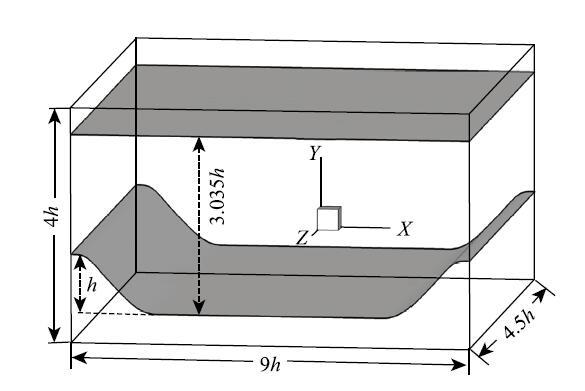图1 周期山状流的几何构型与计算域示意图 Fig. 1 Schematic illustration of the periodic hills configuration and definition of the computational domain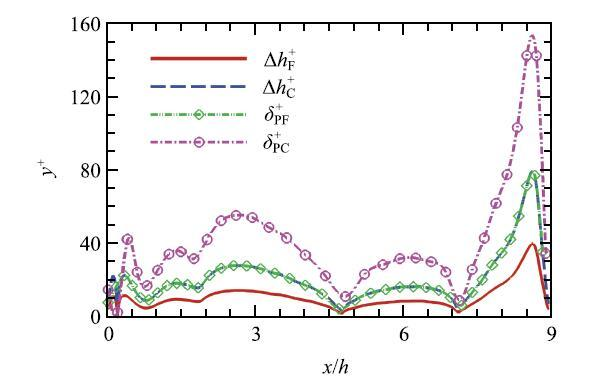图2 周期山状流中以壁面单位无量纲化后的欧拉网格尺度以及匹配面的位置沿下壁面的分布.字符"C"和"F"分别代表粗细两种不同的欧拉网格,字符"P"则表示匹配面上的拉格朗日点 Fig. 2 Non-dimensional resolution of the uniform grids and wall-dista- nces of the matching points along the lower wall for the flow over periodic hills. The letters "C" and "F" represent the coarse and fine grids, respectively, and the letter "P" represents the probe point on the matching surface 图3中给出了周期山状流下壁面的平均压力系数$C_{\rm p}$和平均摩擦力系数$C_{\rm f} $的分布.从图3(a)中的压力系数分布可以看出,除了在山峰后方的分离泡内(${x /}h <4.5)$产生微小偏差外,两种壁模型的预测结果与参考结果整体吻合,尤其是可以准确地捕捉山峰前方(${x/ }h\! >\! 8.0)$伴随流动加速而形成的顺压梯度.不同形式的壁模型在不同网格上的计算结果基本一致,这说明流场压力的计算结果对本文所采用的壁模型形式并不敏感.而对比图3(b)中关于壁面摩擦力的计算结果则可以发现,同一模型在不同网格上的计算结果已经收敛,但是预测结果的准确性则明显依赖于模型形式.NEB模型的计算结果与参考结果吻合较好,尤其是可以准确捕捉山峰前方(${x/ }h \!>\! 8.0)$伴随强压力梯度而形成的摩擦力峰值.而EB模型的计算结果则整体较为平缓,并且在山峰附近严重低估了摩擦力的峰值.该结果表明在壁模型中考虑切向压力梯度项可以明显的改进壁面切应力的计算结果.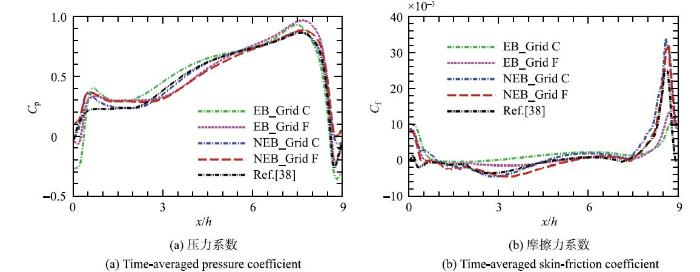图3 周期山状流下壁面的平均压力系数$C_{\rm p} $和平均摩擦力系数$C_{\rm f} $的分布,其中字符"C"和"F"分别代表粗细两种不同的欧拉网格 Fig. 3 Distribution of the time-averaged (a) pressure and (b) skin-friction coefficients along the lower wall for the flow over periodic hills. Grid "C" and "F" represent the coarse and fine grids, respectively 图4中给出了周期山状流不同位置处的流向平均速度剖面的结果.在细网格上,不同形式的壁模型都可以较为准确的预测速度分布.但是NEB模型的计算结果稍微优于EB模型,尤其在靠近上下壁面的区域.NEB模型的优势在粗网格上表现的更为明显.此时,EB模型显著高估了下壁面附近的流向平均速度,同时由于截面等流量的限制高估了上壁面附近的速度.与此相反,NEB模型在粗网格上计算得到的流向平均速度则与细网格上一致并与参考结果非常吻合.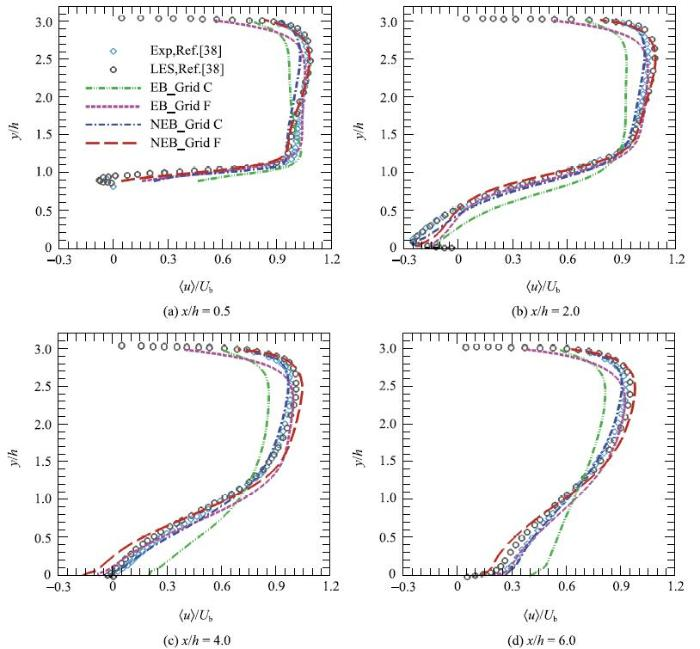图4 周期山状流不同流向位置处的流向平均速度剖面.其中空心圆及菱形符号分别为Breuer 等 的实验及壁面解析的大涡模拟计算结果;红色长虚线以及蓝色点划线分别为NEB模型在细网格及粗网格上的计算结果;紫色短虚线及绿色点点划线分别为EB模型在细网格及粗网格上的计算结果 Fig. 4 The mean streamwise velocity profiles at different locations. The experimental (circles) and wall-resolved LES simulation (diamonds) results by Breuer et al are taken for references. The (red) long dashed lines and (blue) dashed-dot lines are results of the NEB model on fine and coarse grids, respectively. The (purple) short dashed lines and (green) dashed-dot-dot lines are the results of the EB model on fine and coarse grids, respectively 图5中给出了不同流向位置处的法向平均速度的分布结果.可以看出,EB模型即使在细网格上的预测结果在分离点附近($x / h =0.5)$以及回流区内($x / h =2.0)$与参考结果呈现出明显的偏差,粗网格上的结果偏离得更加明显.而NEB模型在不同网格上的预测结果趋于收敛,并且即使在粗网格上的计算结果仍然与参考结果吻合.这表明考虑压力梯度的非平衡壁模型可以有效模拟周期山状流分离点附近以及回流区内的平均流动.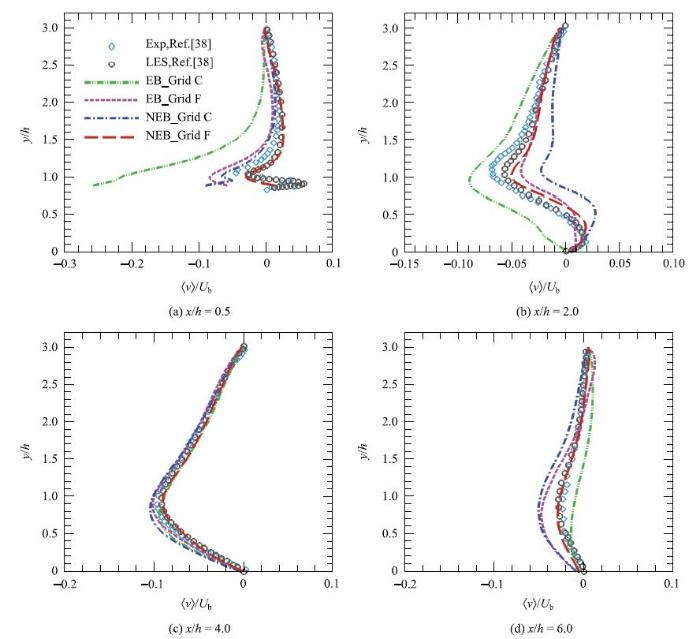图5 周期山状流不同流向位置处的法向平均速度剖面.其中各曲线与符号的代表意义与图4相同 Fig. 5 The mean normal velocity profiles at different locations. Symbols and lines labeled as in Fig.4 不同流向位置处的流向脉动速度方差以及雷诺切应力的分布见图6.不同形式的壁模型计算得到的结果一致.两种模型均可以准确预测流动附着后($x / h \ge4.0)$的雷诺应力分布,但是在分离点附近($x / h = 0.5)$以及回流区($x/ h =2.0)$的分离泡内均低估了速度脉动以及雷诺切应力,并且随着网格的加密结果并没有明显改善.导致这种差异的一种可能原因是NEB模型中没有考虑对流项的影响.下文回转体绕流的算例中存在类似的现象. Larsson等以及Frere等指出由于压力梯度与对流项同量级,在壁模型中不能仅考虑压力梯度项而忽略对流效应.在NEB模型中考虑对流项的影响可能有助于减少误差.另一个可能的原因是在分离点附近和回流区的涡黏系数已不满足关系式(20).Bose和Moin认为回流区内的速度剖面采用线性函数分布即可.壁模型的计算结果与参考结果在分离点和回流区内的这种差异现象,在Temmerman等基于贴体网格以及Chang等基于浸入边界方法的壁面模化大涡模拟中同样存在.在分离点和回流区的下游,EB模型和NEB模型均可以给出较为准确的结果,这是因为此部分区域的脉动量由剪切层的演化所主导,受壁模型的影响较小.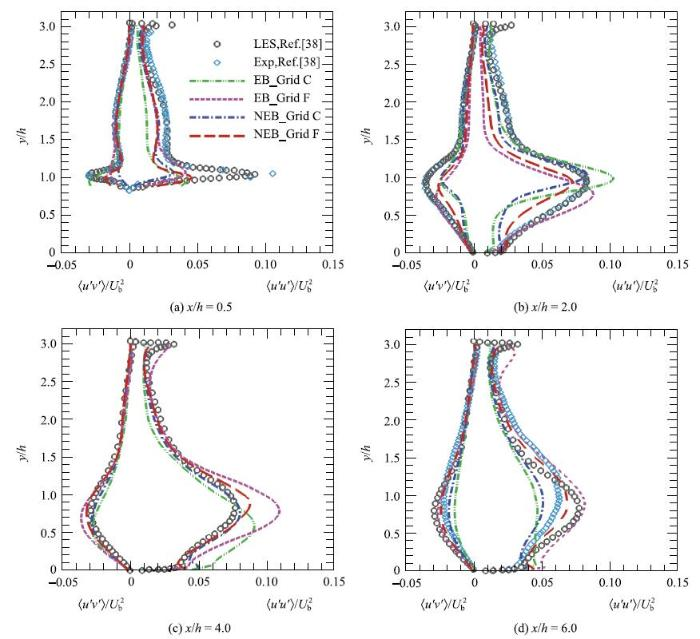图6 周期山状流不同流向位置处的流向速度脉动方差及雷诺切应力的剖面.其中各曲线与符号的代表意义与图4相同 Fig. 6 The resolved fluctuation of the streamwise velocity and the Reynolds shear stress at different locations. Symbols and lines labeled as in Fig.4 图7中给出了粗网格上不同模型计算得到的平均压力分布以及平均流场的流线图.通过与Breuer等的壁面解析大涡模拟的结果对比可以发现,不同模型均可以较好的预测平均压力的分布.但是EB模型预测的分离泡相对更小且更薄,流动分离的较晚同时再附的较早,而NEB模型在粗网格上的计算结果仍然与参考结果保持一致.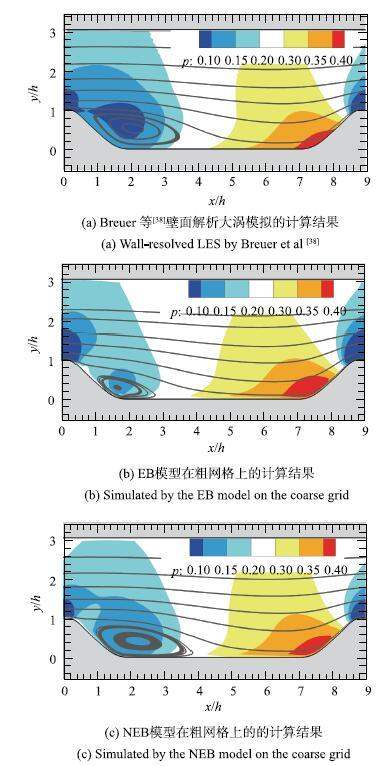图7 周期山状流的平均压力分布以及平均流动的流线图 Fig. 7 Distribution of pressure and streamlines of the mean flow 通过图3$\sim$图7中与参考结果的对比可以看出计算结果对网格尺度以及模型形式的依赖性.相比于EB模型,NEB模型具有明显优势,尤其在计算壁面摩擦力以及预测流动分离及再附方面.EB模型基于平衡边界层假设,该假设在强压力梯度以及分离区内并不成立.而对于NEB模型,简化薄边界层方程的右端项以及涡黏衰减函数均考虑了切向压力梯度的影响,并将其同时用于计算壁面摩擦力以及重构壁面滑移速度,部分反映了近壁流动的非平衡特性.从壁面滑移速度(式(16))和壁面切应力(式(18))的表达式中可以看出,非平衡壁模型的修正项正比于切向压力梯度$\left({\nabla \bar {P}_{\rm m} \cdot {e}_\xi }\right)$和壁面法向距离$\delta _{\rm P} $.因此在强压力梯度区或者网格较粗时,该模型仍然可以准确的预测流动的水动力特征以及平均统计特性. ### 3.2 回转体绕流 为检验浸入边界方法结合滑移速度壁模型模拟工程流动的潜力,本文进一步对高雷诺数回转体绕流进行大涡模拟并分析其水动力特性.该流动的几何构型为无附体的DARPA SUBOFF模型,是研究潜航器绕流的标准模型之一. 轴对称的艇体结构主要由钝型的艏部、圆柱形的中间艇体以及收缩的舰尾组成,其中艇长$L$与截面最大直径$D$的比例约为$8.6$. 回转体绕流的雷诺数(基于艇身长度$L$以及来流速度$U_\infty)$为$1.2\times 10^6$. 其中大涡模拟计算域(流向$\times $法向$\times $展向)为$\left[ { - 2.6D,23.2D} \right]\times \left[ {-4.3D,4.3D} \right] \times \left[ { - 4.3D,4.3D}\right]$. 计算采用的均匀欧拉网格尺度为$\Delta h = D /32$,对应于艇体平行段流动的无量纲壁面单位约为$\Delta h^ + \approx225$. 该算例的计算网格量仅为相同状态下Posa等全附体回转体绕流壁面解析的大涡模拟的1/50.流动的攻角与偏航角均设置为零.在计算域的入口边界给定均匀的来流速度,出口为对流边界条件,侧面边界则设置为自由滑移边界条件.本文分别采用EB与NEB模型来模化回转体近壁区的流场. 本次计算主要关注钝体绕流的平均水动力特性. 根据Posa等对相同状态下全附体的回转体绕流的壁面解析大涡模拟的研究结果,采用如下方法统计平均值：待流动发展完成后,平均量的统计时长为流动由入口流至出口的时间(对应于流动3次流过艇体的时间),对这种轴对称的流动,统计量的计算同时在时间及周向进行平均.本文测试了不同统计时长对结果的影响,如图8所示.当统计时间为一次流过艇体的时间时,艇体表面的平均摩擦力系数已经收敛,因此本文后继关于平均量的统计结果均基于一次流过艇体的时间计算得到.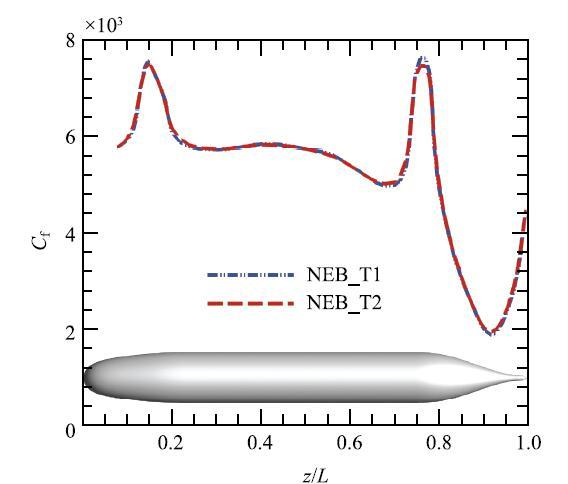图8 NEB模型计算得到的回转体子午线上的平均摩擦系数分布.其中T1的统计时间为流动一次流过计算域的时间,T2为一次流过艇体的时间 Fig. 8 Distributions of the time-averaged skin-friction coefficients at the meridian plane predicted by the NEB model. T1 and T2 are the times of flow past the computational domain and the axisymmetric body, respectively 由NEB模型计算得到的瞬时流场结构如图9所示.图9(a)中给出了流向不同截面位置处以及展向中心截面处的涡量大小的分布云图,揭示了附着于回转体表面的边界层向下游发展的演化过程以及流动尾迹结构的特征.流动经由艏部因几何扩张而加速形成湍流边界层,艇体平行段流动近似为平板边界层流动,而尾部流动则由于几何的收缩而发生减速,并导致边界层变厚.展向中心截面上艇体尾部的涡量分布结果表明流动并未发生明显的分离,这与Huang等的实验观测以及Posa等壁面解析的大涡模拟结果一致.边界层在回转体尾部脱出形成亏损的尾迹并使得涡量集中分布于尾迹截面的中心区域.由Q准则显示的小尺度涡结构见图9(b).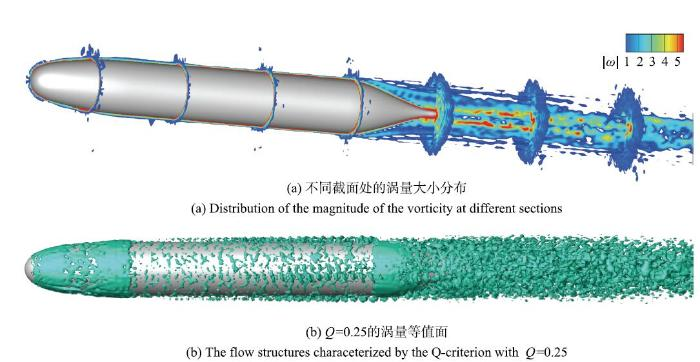图9 由NEB模型计算得到的回转体绕流的瞬时流场结构 Fig. 9 Instantaneous flow structures predicted by the NEB wall model 回转体表面边界层流动的演化历程同样可以由图10中心截面上的平均压力分布来揭示.流动在艏部前缘的驻点区形成高压,向后因边界层加速发展对应于压力向下游的递减.平行段的压力近似维持为常数,使得该区域的近壁流动表现出与零压力梯度平板边界层相似的特性.而尾部则由于几何曲率的变化,边界层减速、变厚并形成明显的逆压梯度.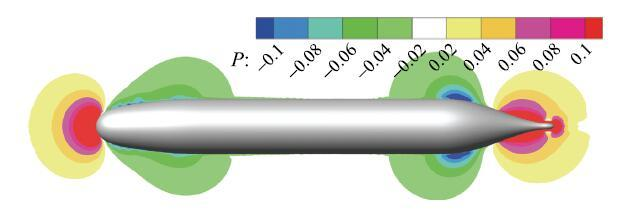图10 由NEB模型计算得到的回转体绕流中心截面($z = 0)$处的平均压力分布云图 Fig. 10 Instantaneous fields of the mean pressure (predicted by the NEB model) at the symmetric plane ($z = 0)$图11中给出了由不同模型计算得到的平均压力系数和摩擦力系数沿壁面子午线的分布.从图11(a)可以看出,两种形式的壁模型均可以准确的预测壁面压力,尤其是能够准确地捕捉回转体艏部及尾部的压力梯度.而对比图11(b)中的计算结果则可以发现两种模型均高估了壁面摩擦力,但是NEB模型的预测结果仍优于EB模型.NEB模型的计算结果与参考结果整体上定性吻合. 尤其是在艏部扩张段($x /D < 0.2)$流动加速的顺压区以及尾部收缩段($x / D>0.75)$流动减速的强逆压梯度区,NEB模型可以较准确地捕捉摩擦力的峰值.而EB模型计算得到的摩擦力系数曲线沿艇体变化比较缓慢,在强压力梯度区的预测结果与参考结果呈现出明显的偏差并且未能捕捉到尾部收缩段摩擦力的峰值.两种形式的壁模型均可以准确的计算压力分布,而非平衡壁模型则可以改进壁面摩擦力的计算结果,这与在周期山状流中观察到的现象一致.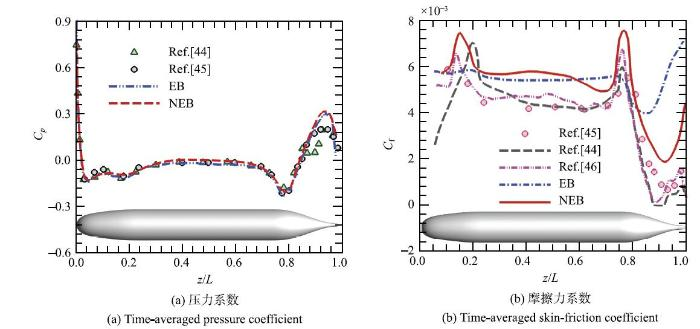图11 回转体绕流中心截面子午线上的平均压力系数$C_{\rm p} $和平均摩擦力系数$C_{\rm f} $的分布 Fig. 11 Distribution of the time-averaged (a) pressure and (b) skin-friction coefficients at the meridian plane 图11(b)中同样给出了直接基于壁面应力构造等效体积力的模拟方法计算结果.可以看出,该方法可以更准确地预测壁面切应力.值得注意的是,该方法采用与滑移速度壁模型相同的方式计算壁面切应力,区别在于与浸入边界方法相结合的方式.滑移速度壁模型通过壁面切应力重构切向滑移速度,法向速度则满足壁面无穿透条件,然后以此构建壁面上的等效体积力.而在直接由壁面切应力构建等效体积力的方法中,壁面切应力直接转化为体积力的形式施加在壁面外附近的代理层上,并隐含了法向速度的影响.根据Bae等的研究工作,法向穿透速度有助于改善流场脉动量的计算结果.另外,在近壁区流动的模型方程中,仅考虑切向压力梯度所引起的非平衡效应.Larsson等以及Frere等则指出,由于压力梯度项与对流项近似平衡,因此薄边界层方程中的压力梯度项与对流项必须同时考虑或忽略.本文下一步的工作将综合考虑上述因素,并对回转体绕流的水动力学特性以及流场尾迹结构特征进行更细致的研究. ## 4 总结与结论 本文采用滑移速度壁模型构建等效体积力,实现了浸入边界方法与壁模型相结合的大涡模拟.分别采用平衡层模型以及考虑切向压力梯度的非平衡壁模型,在周期山状流以及回转体绕流中对比考查了在壁模型中考虑压力梯度的作用. 本文首先在雷诺数为$10~595$的周期山状流中检验了两种壁模型模拟复杂几何边界的湍流分离流动的能力.数值结果表明,流场压力对壁模型的形式并不敏感,但是考虑切向压力梯度可以显著改进壁面摩擦力的计算结果,并且能够准确的预测强压力梯度区以及分离区内流动的平均统计特性.由于平衡边界层假设在强压力梯度以及分离区内并不成立,平衡层模型显著低估了壁面摩擦力,并且难以准确预测该区域内的平均速度剖面.非平衡壁模型在薄边界层方程中考虑了切向压力梯度的影响.在重构壁面滑移速度和计算壁面切应力时的修正项正比于压力梯度和壁面法向距离.因此在流动的强压力梯度区以及网格较粗时,计算得到的平均压力以及摩擦力分布、平均速度剖面以及脉动统计量均与参考的实验和计算结果吻合.在此基础上,本文对雷诺数为$1.2\times10^6\$的回转体绕流进行大涡模拟,以考查该方法应用于高雷诺数壁湍流的适用性.非平衡壁模型可以准确的捕捉典型的流动结构,壁面压力的计算结果与参考结果吻合.与平衡层模型相比,可以更好地预测壁面摩擦力沿艇体的分布,尤其是能够捕捉到艇体尾部的摩擦力峰值.该结果再次证明在壁模型中考虑压力梯度可以改进壁面摩擦力的计算结果.

The authors have declared that no competing interests exist.

## 参考文献 原文顺序 文献年度倒序 文中引用次数倒序 被引期刊影响因子

  林孟达, 崔桂香, 张兆顺等. 飞机尾涡演变及快速预测的大涡模拟研究. 力学学报, 2017, 49(6): 1185-1200 随着我国人民生活水平的提高，航空运输的重要性与日俱增，航班延误问题也日益严重.尾流间隔（保障后机不受前机尾流影响的最小安全间隔）是制约机场效率的关键因素.针对这一工程应用问题，采用大涡模拟方法研究飞机尾涡在大气中的演变特性.研究工作首先发展了飞机尾涡演变的大涡模拟方法，将自适应网格技术应用于飞机尾涡演变的大涡模拟，大幅减少所需的网格量，提高计算效率.提出了升力面尾涡生成方法，在不增加计算量的情况下实现了尾涡卷起过程和远场衰减的组合模拟.在系列算例分析研究基础上，创建了基于大涡模拟计算结果的尾流间隔快速预测系统.该系统可以根据实时大气风场和进出港的前后飞机机型，快速预测并输出所需的尾流间隔.经过与场地测试数据比较表明，在北京市2014年的平均风速条件下，本系统预测的尾流间隔可在现有标准基础上缩减7%~50%，能够有效提高机场容量. (Lin Mengda, Cui Guixiang, Zhang Zhaoshun, et al.Large eddy simulation on the evolution and the fast-time prediction of aircraft wake vortices. Chinese Journal of Theoretical and Applied Mechanics, 2017, 49(6): 1185-1200 (in Chinese)) 随着我国人民生活水平的提高，航空运输的重要性与日俱增，航班延误问题也日益严重.尾流间隔（保障后机不受前机尾流影响的最小安全间隔）是制约机场效率的关键因素.针对这一工程应用问题，采用大涡模拟方法研究飞机尾涡在大气中的演变特性.研究工作首先发展了飞机尾涡演变的大涡模拟方法，将自适应网格技术应用于飞机尾涡演变的大涡模拟，大幅减少所需的网格量，提高计算效率.提出了升力面尾涡生成方法，在不增加计算量的情况下实现了尾涡卷起过程和远场衰减的组合模拟.在系列算例分析研究基础上，创建了基于大涡模拟计算结果的尾流间隔快速预测系统.该系统可以根据实时大气风场和进出港的前后飞机机型，快速预测并输出所需的尾流间隔.经过与场地测试数据比较表明，在北京市2014年的平均风速条件下，本系统预测的尾流间隔可在现有标准基础上缩减7%~50%，能够有效提高机场容量.  冯峰, 郭力, 王强. 高亚声速湍流喷流气动噪声数值分析. 力学学报, 2016, 48(5): 1049-1060

(Feng Feng, Guo Li, Wang Qiang.Numerical investigation of noise of a high subsonic turbulent jet. Chinese Journal of Theoretical and Applied Mechanics, 2016, 48(5): 1049-1060 (in Chinese))

 周磊, 解茂昭, 罗开红等. 大涡模拟在内燃机中应用的研究进展. 力学学报, 2013, 45(4): 467-482

(Zhou Lei, Xie Maozhao, Luo Kaihong, et al.Large eddy simulation for internal combustion engines: progress and prospects. Chinese Journal of Theoretical and Applied Mechanics, 2013, 45(4): 467-482 (in Chinese))〈〉